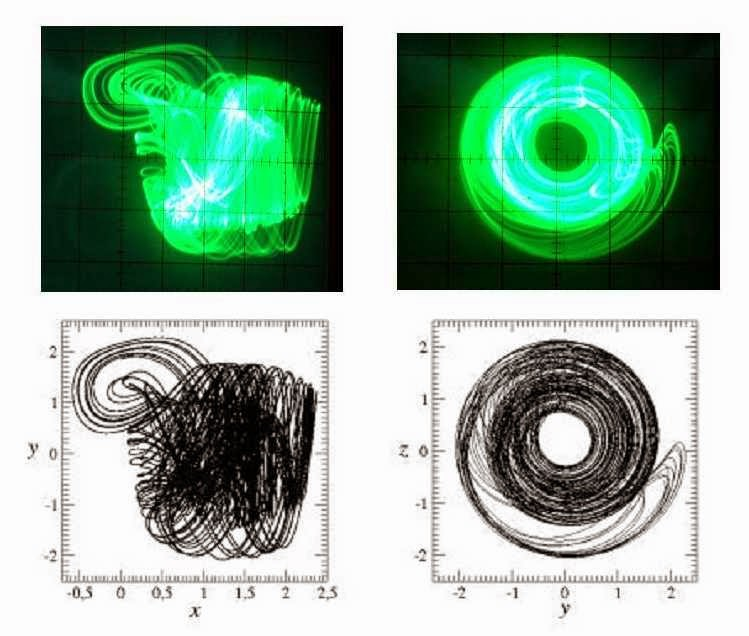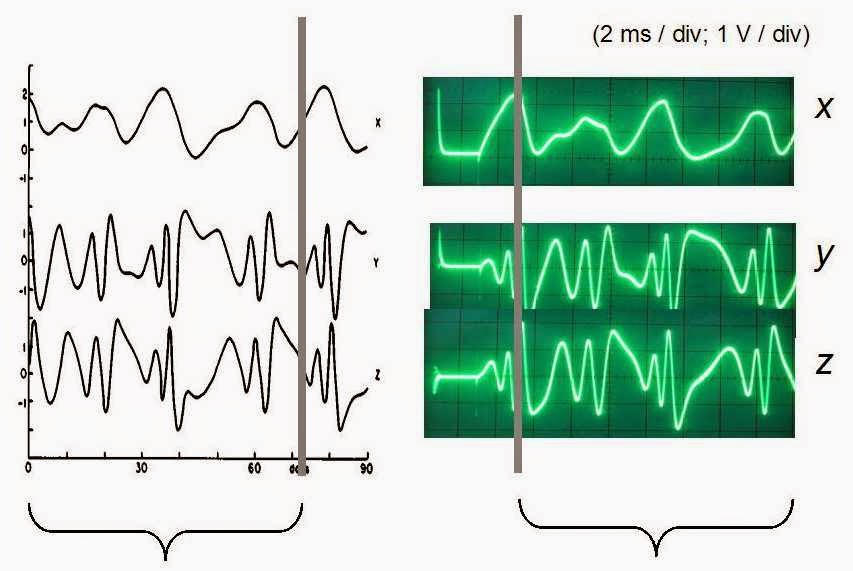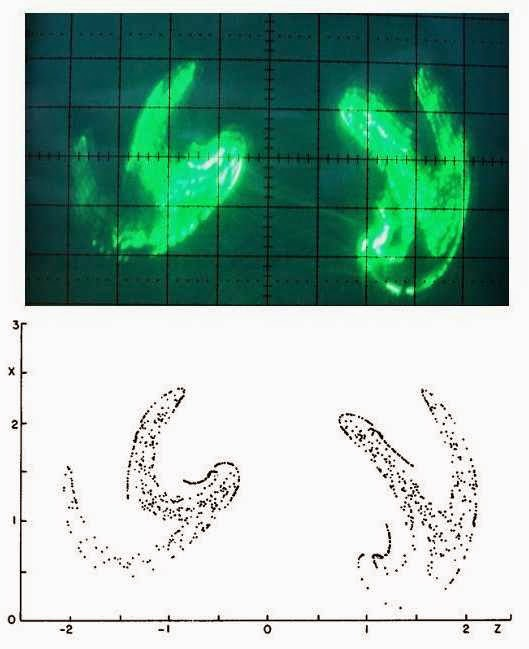## Saturday, February 21, 2015

### Lorenz 84 System

A model for the long-term atmospheric circulation closest to the equator (the Hadley cell), proposed by Lorenz  in 1984, is given by:

I really like this sort of pragmatic physics - i.e. simple low order models which still retain the importance of what's actually going on in Nature. What amazes me also is how easy / quick it is to sketch out how to patch these up on the analog computer:

Adopting a = 0.25, b = 4.0, F = 8.0 and G = 1.0 (as used by Lorenz in reference ), yields the following attractors. These show nice agreement with those given in :Lorenz 84 System Attractor - lower plots from . Upper plots 1 V / div except left plot X axis at 0.5 V / div.
The following plots show the variations of x, y and z as per reference , Figure 5. It seems that the initial conditions used by Lorenz are different to mine (I start off with x = y = z = 0); unfortunately I do not know what Lorenz used as initial conditions - the left hand side of his Figure 5 suggests values other than zero. Interestingly, my x, y and z build up into what seems to be Lorenz's values after a period of time - represented by the right hand vertical bar below:Variation of x, y and z. Left hand side is Figure 5 from reference .
The braced regions look similar - about 72 days of Figure 5 corresponding to 14.5 ms of the oscilloscope plots.

Finally, I had a go at producing a plot of where the attractor intersects the y = 0 plane. To do this I connected the output of the integrator which dealt with the 2nd (i.e. dy/dt) equation to one of the two x = y functions on the computer. If x = y then the scope's z-axis is given -10 V else it gets +10 V. On the Tektronix 465 the latter decreases the intensity of the beam, the former brightens it. The result was pretty impressive - and compares nicely with Figure 7 of reference . The distinctive (Cantor-set) gaps between 'fingers' is evident.Intersection with y = 0 plane; lower plot from reference . Upper plot 0.5 V / div.
(The scope's brightness is not truly on / off - hence some 'ghost' lines showing through.) Nice that I pick up (some of) the more solid (brighter) regions around z = 1, x = 0.5 V.

Here's what the computer looked like for this problem (note sides / back still need fitting):

...and the xy-projection of the attractor drawn out at slow speed on the plotter (x-axis at 0.2 V / cm and y-axis at 0.5 V / cm):
References

 E. N. Lorenz, Irregularity: a fundamental property of the atmosphere, Tellus A, 36, 98-110, 1984.

 Christophe Letellier, (Lorenz) 1984 A 3D model for global atmospheric circulation, Université & INSA de Rouen, 3rd March 2008.